# Learning Objectives

### Learning Objectives

By the end of this section, you will be able to do the following:

• Interpret circuit diagrams with parallel resistors
• Calculate equivalent resistance of resistor combinations containing series and parallel resistors
 in parallel

# Resistors in Parallel

### Resistors in Parallel

In the previous section, we learned that resistors in series are resistors that are connected one after the other. If we instead combine resistors by connecting them next to each other, as shown in Figure 19.19, then the resistors are said to be connected in parallel. Resistors are in parallel when both ends of each resistor are connected directly together.

Note that the tops of the resistors are all connected to the same wire, so the voltage at the top of the each resistor is the same. Likewise, the bottoms of the resistors are all connected to the same wire, so the voltage at the bottom of each resistor is the same. This means that the voltage drop across each resistor is the same. In this case, the voltage drop is the voltage rating V of the battery, because the top and bottom wires connect to the positive and negative terminals of the battery, respectively.

Although the voltage drop across each resistor is the same, we cannot say the same for the current running through each resistor. Thus, $I1, I2, and I3I1, I2, and I3$ are not necessarily the same, because the resistors $R1, R2, and R3R1, R2, and R3$ do not necessarily have the same resistance.

Note that the three resistors in Figure 19.19 provide three different paths through which the current can flow. This means that the equivalent resistance for these three resistors must be less than the smallest of the three resistors. To understand this, imagine that the smallest resistor is the only path through which the current can flow. Now add on the alternate paths by connecting other resistors in parallel. Because the current has more paths to go through, the overall resistance (i.e., the equivalent resistance) will decrease. Therefore, the equivalent resistance must be less than the smallest resistance of the parallel resistors.

Figure 19.19 The left circuit diagram shows three resistors in parallel. The voltage V of the battery is applied across all three resistors. The currents that flow through each branch are not necessarily equal. The right circuit diagram shows an equivalent resistance that replaces the three parallel resistors.

To find the equivalent resistance $RequivRequiv$ of the three resistors $R1, R2, and R3R1, R2, and R3$, we apply Ohm’s law to each resistor. Because the voltage drop across each resistor is V, we obtain

19.21$V=I1R1, V=I2R2, V=I3R3V=I1R1, V=I2R2, V=I3R3$

or

19.22$I1=VR1, I2=VR2, I3=VR3.I1=VR1, I2=VR2, I3=VR3.$

We also know from conservation of charge that the three currents $I1, I2, and I3I1, I2, and I3$ must add up to give the current I that goes through the battery. If this were not true, current would have to be mysteriously created or destroyed somewhere in the circuit, which is physically impossible. Thus, we have

19.23$I=I1+I2+I3.I=I1+I2+I3.$

Inserting the expressions for $I1, I2, and I3I1, I2, and I3$ into this equation gives

19.24$I=VR1+VR2+VR3=V(1R1+1R2+1R3)I=VR1+VR2+VR3=V(1R1+1R2+1R3)$

or

19.25$V=I(11/R1+1/R2+1/R3).V=I(11/R1+1/R2+1/R3).$

This formula is just Ohm’s law, with the factor in parentheses being the equivalent resistance.

19.26$V=I(11/R1+1/R2+1/R3)=IRequiv.V=I(11/R1+1/R2+1/R3)=IRequiv.$

Thus, the equivalent resistance for three resistors in parallel is

19.27$Requiv=11/R1+1/R2+1/R3.Requiv=11/R1+1/R2+1/R3.$

The same logic works for any number of resistors in parallel, so the general form of the equation that gives the equivalent resistance of N resistors connected in parallel is

19.28 $Requiv=11/R1+1/R2+⋯+1/RN.Requiv=11/R1+1/R2+⋯+1/RN.$

### Worked Example

#### Find the Current through Parallel Resistors

The three circuits below are equivalent. If the voltage rating of the battery is $Vbattery=3 VVbattery=3 V$, what is the equivalent resistance of the circuit and what current runs through the circuit?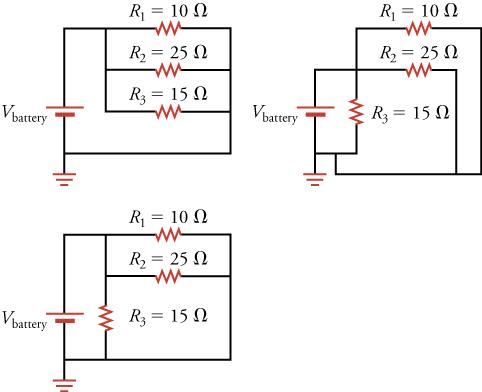### STRATEGY

The three resistors are connected in parallel and the voltage drop across them is Vbattery. Thus, we can apply the equation for the equivalent resistance of resistors in parallel, which takes the form

19.29$Requiv=11/R1+1/R2+1/R3.Requiv=11/R1+1/R2+1/R3.$

The circuit with the equivalent resistance is shown below. Once we know the equivalent resistance, we can use Ohm’s law to find the current in the circuit.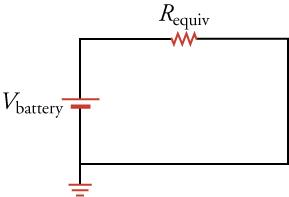Solution

Inserting the given values for the resistance into the equation for equivalent resistance gives

19.30$Requiv=11/R1+1/R2+1/R3=11/10 Ω+1/25 Ω+1/15 Ω=4.84 Ω.Requiv=11/R1+1/R2+1/R3=11/10 Ω+1/25 Ω+1/15 Ω=4.84 Ω.$

The current through the circuit is thus

19.31$V=IRI=VR=3 V4.84 Ω=0.62 A.V=IRI=VR=3 V4.84 Ω=0.62 A.$
Discussion

Although 0.62 A flows through the entire circuit, note that this current does not flow through each resistor. However, because electric charge must be conserved in a circuit, the sum of the currents going through each branch of the circuit must add up to the current going through the battery. In other words, we cannot magically create charge somewhere in the circuit and add this new charge to the current. Let’s check this reasoning by using Ohm’s law to find the current through each resistor.

19.32$I1=VR1=3 V10 Ω=0.30 AI2=VR2=3 V25 Ω=0.12 AI3=VR3=3 V15 Ω=0.20 AI1=VR1=3 V10 Ω=0.30 AI2=VR2=3 V25 Ω=0.12 AI3=VR3=3 V15 Ω=0.20 A$

As expected, these currents add up to give 0.62 A, which is the total current found going through the equivalent resistor. Also, note that the smallest resistor has the largest current flowing through it, and vice versa.

### Worked Example

#### Reasoning with Parallel Resistors

Without doing any calculation, what is the equivalent resistance of three identical resistors R in parallel?

### STRATEGY

Three identical resistors R in parallel make three identical paths through which the current can flow. Thus, it is three times easier for the current to flow through these resistors than to flow through a single one of them.

Solution

If it is three times easier to flow through three identical resistors R than to flow through a single one of them, the equivalent resistance must be three times less: R/3.

Discussion

Let’s check our reasoning by calculating the equivalent resistance of three identical resistors R in parallel. The equation for the equivalent resistance of resistors in parallel gives

19.33$Requiv=11/R+1/R+1/R=13/R=R3.Requiv=11/R+1/R+1/R=13/R=R3.$

Thus, our reasoning was correct. In general, when more paths are available through which the current can flow, the equivalent resistance decreases. For example, if we have identical resistors R in parallel, the equivalent resistance would be R/10.

# Practice Problems

### Practice Problems

Three resistors, 10, 20, and 30 Ω, are connected in parallel. What is the equivalent resistance?

1. The equivalent resistance is 5.5 Ω
2. The equivalent resistance is 60 Ω
3. The equivalent resistance is 6 × 103 Ω
4. The equivalent resistance is 6 × 104 Ω
If a $5-V$ drop occurs across $R1$, and $R1$ is connected in parallel to $R2$, what is the voltage drop across $R2$?
1. Voltage drop across is $0V$.
2. Voltage drop across is $2.5V$.
3. Voltage drop across is $5V$.
4. Voltage drop across is $10V$.

# Resistors in Parallel and in Series

### Resistors in Parallel and in Series

More complex connections of resistors are sometimes just combinations of series and parallel. Combinations of series and parallel resistors can be reduced to a single equivalent resistance by using the technique illustrated in Figure 19.20. Various parts are identified as either series or parallel, reduced to their equivalents, and further reduced until a single resistance is left. The process is more time consuming than difficult.

Figure 19.20 This combination of seven resistors has both series and parallel parts. Each is identified and reduced to an equivalent resistance, and these are further reduced until a single equivalent resistance is reached.

Let’s work through the four steps in Figure 19.20 to reduce the seven resistors to a single equivalent resistor. To avoid distracting algebra, we’ll assume each resistor is 10 $ΩΩ$. In step 1, we reduce the two sets of parallel resistors circled by the blue dashed loop. The upper set has three resistors in parallel and will be reduced to a single equivalent resistor $RP1RP1$. The lower set has two resistors in parallel and will be reduced to a single equivalent resistor $RP2RP2$. Using the equation for the equivalent resistance of resistors in parallel, we obtain

19.34$RP1=11/R2+1/R3+1/R4=11/10 Ω+1/10 Ω+1/10 Ω=103 ΩRP2=11/R5+1/R6=11/10 Ω+1/10 Ω=5 Ω.RP1=11/R2+1/R3+1/R4=11/10 Ω+1/10 Ω+1/10 Ω=103 ΩRP2=11/R5+1/R6=11/10 Ω+1/10 Ω=5 Ω.$

These two equivalent resistances are encircled by the red dashed loop following step 1. They are in series, so we can use the equation for the equivalent resistance of resistors in series to reduce them to a single equivalent resistance $RS1RS1$. This is done in step 2, with the result being

19.35$RS1=RP1+RP2=103 Ω+5 Ω=253 Ω.RS1=RP1+RP2=103 Ω+5 Ω=253 Ω.$

The equivalent resistor $RS1RS1$ appears in the green dashed loop following step 2. This resistor is in parallel with resistor $R7R7$, so the pair can be replaced by the equivalent resistor $RP3RP3$, which is given by

19.36$RP3=11/RS1+1/R7=13/25 Ω+1/10 Ω=5011 Ω.RP3=11/RS1+1/R7=13/25 Ω+1/10 Ω=5011 Ω.$

This is done in step 3. The resistor $RP3RP3$ is in series with the resistor $R1R1$, as shown in the purple dashed loop following step 3. These two resistors are combined in the final step to form the final equivalent resistor $RequivRequiv$, which is

19.37$Requiv=R1+RP3=10 Ω+5011 Ω=16011 Ω.Requiv=R1+RP3=10 Ω+5011 Ω=16011 Ω.$

Thus, the entire combination of seven resistors may be replaced by a single resistor with a resistance of about 14.5 $ΩΩ$.

That was a lot of work, and you might be asking why we do it. It’s important for us to know the equivalent resistance of the entire circuit so that we can calculate the current flowing through the circuit. Ohm’s law tells us that the current flowing through a circuit depends on the resistance of the circuit and the voltage across the circuit. But to know the current, we must first know the equivalent resistance.

Here is a general approach to find the equivalent resistor for any arbitrary combination of resistors:

1. Identify a group of resistors that are only in parallel or only in series.
2. For resistors in series, use the equation for the equivalent resistance of resistors in series to reduce them to a single equivalent resistance. For resistors in parallel, use the equation for the equivalent resistance of resistors in parallel to reduce them to a single equivalent resistance.
3. Draw a new circuit diagram with the resistors from step 1 replaced by their equivalent resistor.
4. If more than one resistor remains in the circuit, return to step 1 and repeat. Otherwise, you are finished.

### Fun In Physics

#### Robot

Robots have captured our collective imagination for over a century. Now, this dream of creating clever machines to do our dirty work, or sometimes just to keep us company, is becoming a reality. Robotics has become a huge field of research and development, with some technology already being commercialized. Think of the small autonomous vacuum cleaners, for example.

Figure 19.21 shows just a few of the multitude of different forms robots can take. The most advanced humanoid robots can walk, pour drinks, even dance (albeit not very gracefully). Other robots are bio-inspired, such as the dogbot shown in the middle photograph of Figure 19.21. This robot can carry hundreds of pounds of load over rough terrain. The photograph on the right in Figure 19.21 shows the inner workings of an M-block, developed by the Massachusetts Institute of Technology. These simple-looking blocks contain inertial wheels and electromagnets that allow them to spin and flip into the air and snap together in a variety of shapes. By communicating wirelessly between themselves, they self-assemble into a variety of shapes, such as desks, chairs, and someday maybe even buildings.

All robots involve an immense amount of physics and engineering. The simple act of pouring a drink has only recently been mastered by robots, after over 30 years of research and development! The balance and timing that we humans take for granted is in fact a very tricky act to follow, requiring excellent balance, dexterity, and feedback. To master this requires sensors to detect balance, computing power to analyze the data and communicate the appropriate compensating actions, and joints and actuators to implement the required actions.

In addition to sensing gravity or acceleration, robots can contain multiple different sensors to detect light, sound, temperature, smell, taste, etc. These devices are all based on the physical principles that you are studying in this text. For example, the optics used for robotic vision are similar to those used in your digital cameras: pixelated semiconducting detectors in which light is converted into electrical signals. To detect temperature, simple thermistors may be used, which are resistors whose resistance changes depending on temperature.

Building a robot today is much less arduous than it was a few years ago. Numerous companies now offer kits for building robots. These range in complexity something suitable for elementary school children to something that would challenge the best professional engineers. If interested, you may find these easily on the Internet and start making your own robot today.

Figure 19.21 Robots come in many shapes and sizes, from the classic humanoid type to dogbots to small cubes that self-assemble to perform a variety of tasks.

### Watch Physics

#### Resistors in Parallel

This video shows a lecturer discussing a simple circuit with a battery and a pair of resistors in parallel. He emphasizes that electrons flow in the direction opposite to that of the positive current and also makes use of the fact that the voltage is the same at all points on an ideal wire. The derivation is quite similar to what is done in this text, but the lecturer goes through it well, explaining each step.

Grasp Check

True or false—In a circuit diagram, we can assume that the voltage is the same at every point in a given wire.

1. false
2. true

### Watch Physics

#### Resistors in Series and in Parallel

This video shows how to calculate the equivalent resistance of a circuit containing resistors in parallel and in series. The lecturer uses the same approach as outlined above for finding the equivalent resistance.

Grasp Check

Imagine connected N identical resistors in parallel. Each resistor has a resistance of R. What is the equivalent resistance for this group of parallel resistors?

1. The equivalent resistance is (R)N.
2. The equivalent resistance is NR.
3. The equivalent resistance is $RN.RN.$
4. The equivalent resistance is $NR.NR.$

### Worked Example

#### Find the Current through a Complex Resistor Circuit

The battery in the circuit below has a voltage rating of 10 V. What current flows through the circuit and in what direction?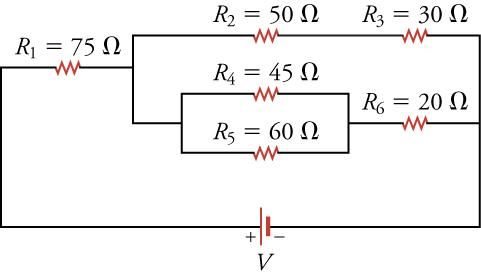### STRATEGY

Apply the strategy for finding equivalent resistance to replace all the resistors with a single equivalent resistance, then use Ohm’s law to find the current through the equivalent resistor.

Solution

The resistor combination $R4R4$ and $R5R5$ can be reduced to an equivalent resistance of

19.38$RP1=11/R4+1/R5=11/45 Ω+1/60 Ω=25.71 ΩR.RP1=11/R4+1/R5=11/45 Ω+1/60 Ω=25.71 ΩR.$

Replacing $R4R4$ and $R5R5$ with this equivalent resistance gives the circuit below.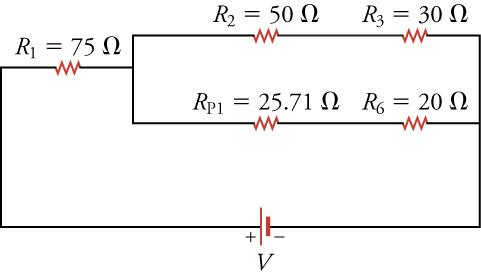We now replace the two upper resistors $R2R2$ and $R3R3$ by the equivalent resistor $RS1RS1$ and the two lower resistors $RP1RP1$ and $R6R6$ by their equivalent resistor $RS2RS2$. These resistors are in series, so we add them together to find the equivalent resistance.

19.39$RS1=R2+R3=50 Ω+30 Ω=80 ΩRS2=RP1+R6=25.71 Ω+20 Ω=45.71 ΩRS1=R2+R3=50 Ω+30 Ω=80 ΩRS2=RP1+R6=25.71 Ω+20 Ω=45.71 Ω$

Replacing the relevant resistors with their equivalent resistor gives the circuit below.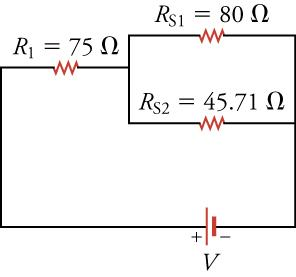Now replace the two resistors , which are in parallel, with their equivalent resistor $RP2RP2$. The resistance of $RP2RP2$ is

19.40$RP2=11/RS1+1/RS2=11/80 Ω+1/45.71 Ω=29.09 Ω.RP2=11/RS1+1/RS2=11/80 Ω+1/45.71 Ω=29.09 Ω.$

Updating the circuit diagram by replacing with this equivalent resistance gives the circuit below.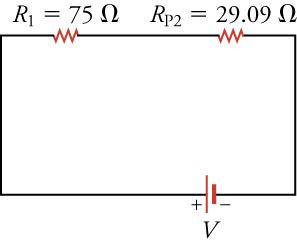Finally, we combine resistors , which are in series. The equivalent resistance is $RS3=R1+RP2=75 Ω+29.09 Ω=104.09 Ω.RS3=R1+RP2=75 Ω+29.09 Ω=104.09 Ω.$ The final circuit is shown below.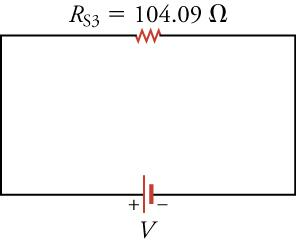We now use Ohm’s law to find the current through the circuit.

19.41$V=IRS3I=VRS3=10 V104.09 Ω=0.096 AV=IRS3I=VRS3=10 V104.09 Ω=0.096 A$

The current goes from the positive terminal of the battery to the negative terminal of the battery, so it flows clockwise in this circuit.

Discussion

This calculation may seem rather long, but with a little practice, you can combine some steps. Note also that extra significant digits were carried through the calculation. Only at the end was the final result rounded to two significant digits.

### Worked Example

#### Strange-Looking Circuit Diagrams

Occasionally, you may encounter circuit diagrams that are not drawn very neatly, such as the diagram shown below. This circuit diagram looks more like how a real circuit might appear on the lab bench. What is the equivalent resistance for the resistors in this diagram, assuming each resistor is 10 $ΩΩ$ and the voltage rating of the battery is 12 V.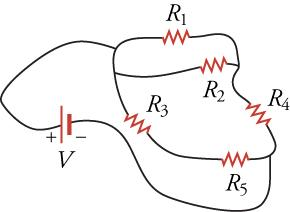### STRATEGY

Let’s redraw this circuit diagram to make it clearer. Then we’ll apply the strategy outlined above to calculate the equivalent resistance.

Solution

To redraw the diagram, consider the figure below. In the upper circuit, the blue resistors constitute a path from the positive terminal of the battery to the negative terminal. In parallel with this circuit are the red resistors, which constitute another path from the positive to negative terminal of the battery. The blue and red paths are shown more cleanly drawn in the lower circuit diagram. Note that, in both the upper and lower circuit diagrams, the blue and red paths connect the positive terminal of the battery to the negative terminal of the battery.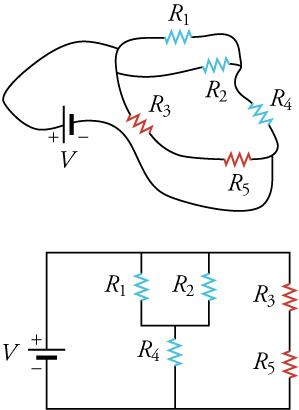Now it is easier to see that are in parallel, and the parallel combination is in series with $R4R4$. This combination in turn is in parallel with the series combination of . First, we calculate the blue branch, which contains . The equivalent resistance is

19.42$Rblue=11/R1+1/R2+R4=11/10 Ω+1/10 Ω+10 Ω=15 Ω.Rblue=11/R1+1/R2+R4=11/10 Ω+1/10 Ω+10 Ω=15 Ω.$

where we show the contribution from the parallel combination of resistors and from the series combination of resistors. We now calculate the equivalent resistance of the red branch, which is

19.43$Rred=R3+R5=10 Ω+10 Ω=20 Ω.Rred=R3+R5=10 Ω+10 Ω=20 Ω.$

Inserting these equivalent resistors into the circuit gives the circuit below.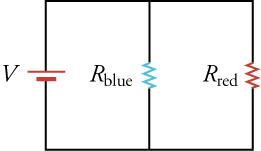These two resistors are in parallel, so they can be replaced by a single equivalent resistor with a resistance of

19.44$Requiv=11/Rblue+1/Rred=11/15 Ω+1/20 Ω=8.6 Ω.Requiv=11/Rblue+1/Rred=11/15 Ω+1/20 Ω=8.6 Ω.$

The final equivalent circuit is show below.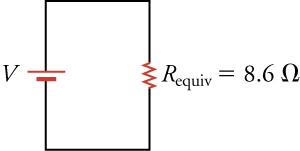Discussion

Finding the equivalent resistance was easier with a clear circuit diagram. This is why we try to make clear circuit diagrams, where the resistors in parallel are lined up parallel to each other and at the same horizontal position on the diagram.

We can now use Ohm’s law to find the current going through each branch to this circuit. Consider the circuit diagram with $RblueRblue$ and $RredRred$. The voltage across each of these branches is 12 V (i.e., the voltage rating of the battery). The current in the blue branch is

19.45$Iblue=VRblue=12 V15 Ω=0.80 A.Iblue=VRblue=12 V15 Ω=0.80 A.$

The current across the red branch is

19.46$Ired=VRred=12 V20 Ω=0.60 A.Ired=VRred=12 V20 Ω=0.60 A.$

The current going through the battery must be the sum of these two currents (can you see why?), or 1.4 A.

# Practice Problems

### Practice Problems

What is the formula for the equivalent resistance of two parallel resistors with resistance R1 and R2?

1. Equivalent resistance of two parallel resistors $Reqv=R1+R2Reqv=R1+R2$
2. Equivalent resistance of two parallel resistors $Reqv=R1×R2Reqv=R1×R2$
3. Equivalent resistance of two parallel resistors $Reqv=R1-R2Reqv=R1-R2$
4. Equivalent resistance of two parallel resistors $Reqv=11/R1+1/R2Reqv=11/R1+1/R2$
Figure 19.22

What is the equivalent resistance for the two resistors shown below?

1. The equivalent resistance is 20 Ω
2. The equivalent resistance is 21 Ω
3. The equivalent resistance is 90 Ω
4. The equivalent resistance is 1,925 Ω

Exercise 6

The voltage drop across parallel resistors is ________.

1. the same for all resistors
2. greater for the larger resistors
3. less for the larger resistors
4. greater for the smaller resistors
Exercise 7

Consider a circuit of parallel resistors. The smallest resistor is 25 Ω . What is the upper limit of the equivalent resistance?

1. The upper limit of the equivalent resistance is 2.5 Ω.
2. The upper limit of the equivalent resistance is 25 Ω.
3. The upper limit of the equivalent resistance is 100 Ω.
4. There is no upper limit.